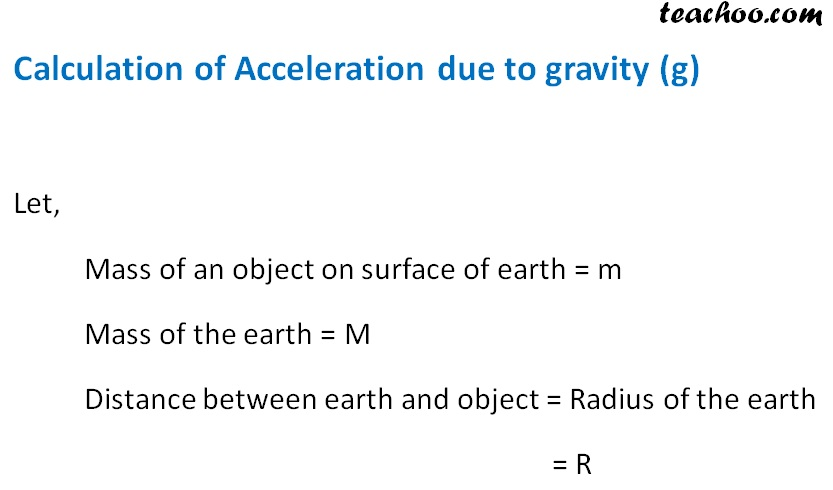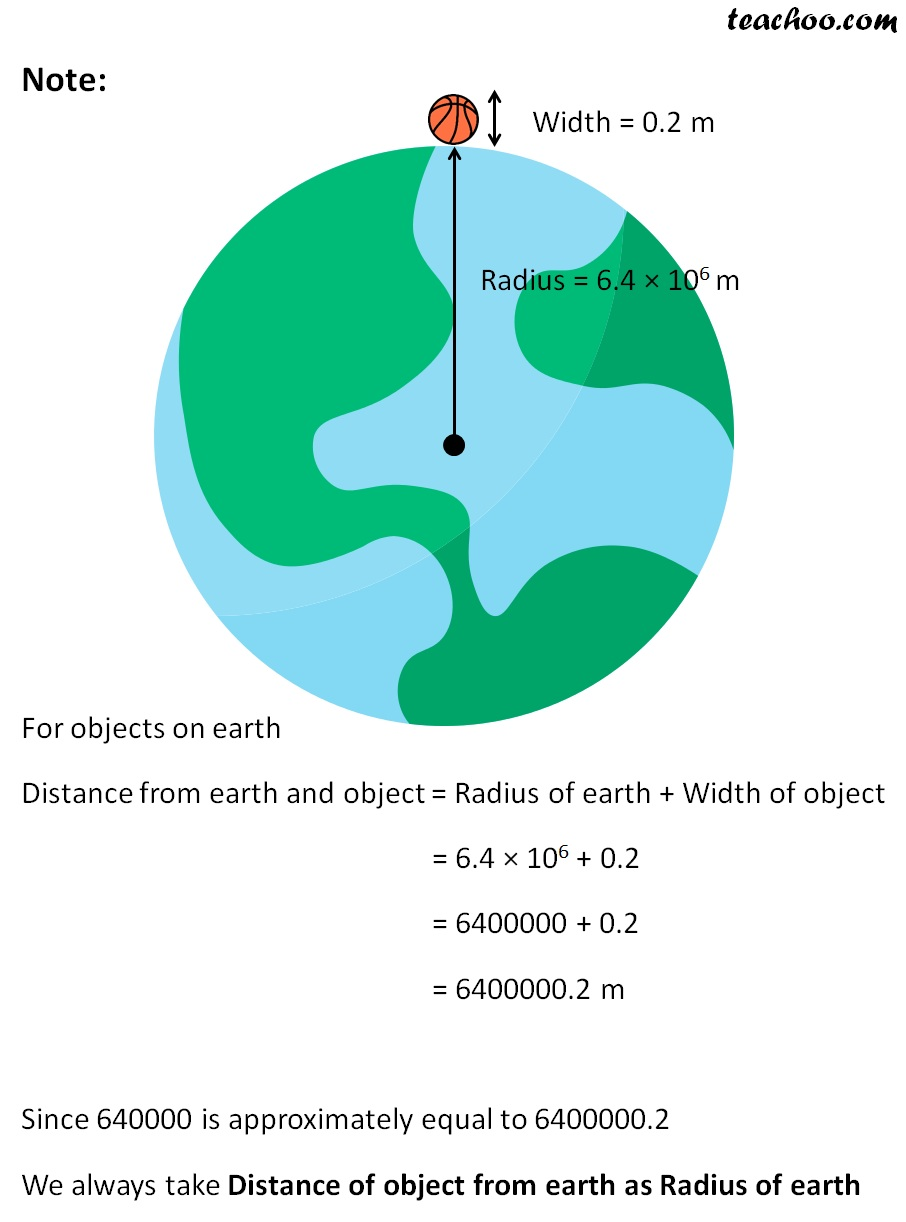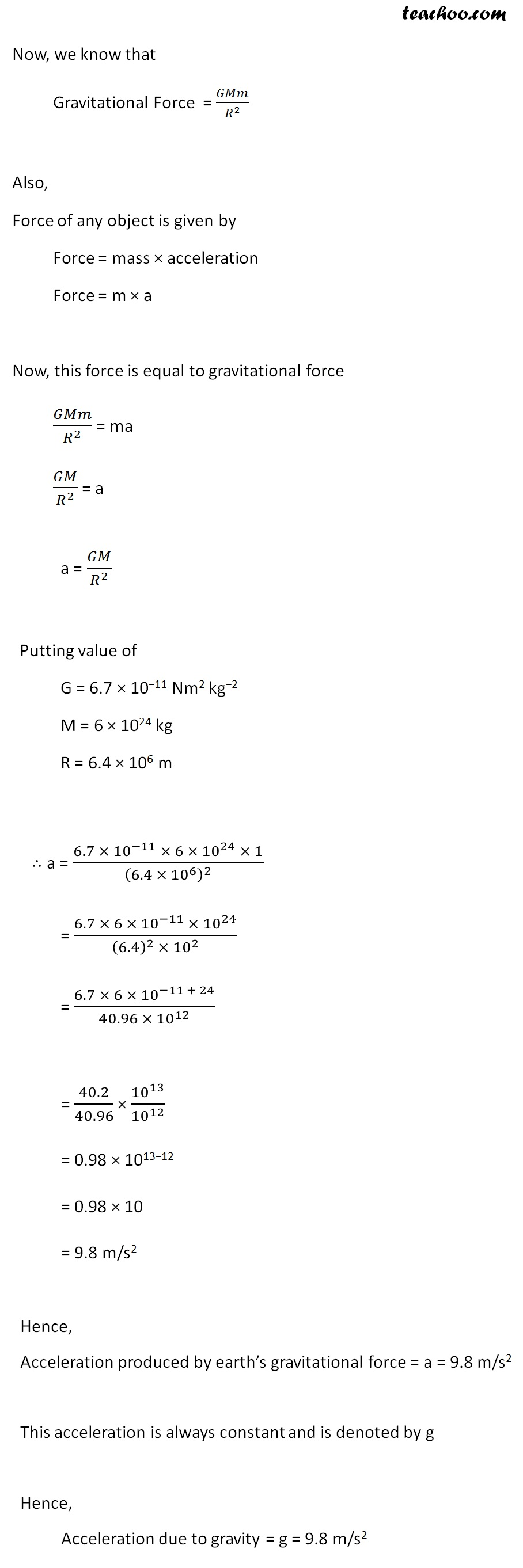Concepts

Class 9
Chapter 10 Class 9 - Gravitation

## What is free fall

When a body or object

falls towards earth

due to gravitational force of earth

and without any other force acting on it

It is called free fall

## When a body falls from sky to earth, does it fall with uniform speed?

No

It does not fall with uniform speed

Its speed actually increases as it falls on earth

This is due to acceleration due to gravity

## What is the meaning of Acceleration due to Gravity?

When a body falls on earth

a uniform acceleration is produced (velocity or speed increases)

due to gravitational force of the earth

This is called acceleration due to gravity

This Acceleration is denoted by g

g = 9.8 m/s 2

Similarly,if a body is thrown upwards

a uniform retardation is produced (velocity or speed decreases)

This is denoted by

g = -9.8 m/s 2

How to Calculate Acceleration due to Gravity

We know that## Acceleration does not depend upon Mass of Body

If 2 objects are falling, even if they have different mass, still they will fall with same acceleration of 9.8m/s 2

Explanation

We now know that

g = G x M/r 2

here

G = Gravitational Constant

M = Mass of earth

All these are constant and do not change

So we can say that

Value of g remains constant

It does not depend upon mass of body falling

If 2 objects are falling, even if they have different mass, still they will have same acceleration

## Why does stone fall on earth quickly but a paper takes time to fall?

It is because paper faces more air resistance compared to stone

Paper has lesser mass compared to its Surface area so it faces more air resistance and hence fall slowly

Stone has more mass compared to its surface area so it faces lesser air resistance while falling down and hence fall quickly

Note

Acceleration due to gravity does not depend upon mass of object

If we remove air and then make both paper and stone fall, both will fall with same acceleration of 9.8 m /s 2

it is because of air resistance that stone falls faster but paper takes time to fall

## Questions

Q 1 Page 136 - What do you mean by free fall?

Q 2 Page 136 - What do you mean by acceleration due to gravity

NCERT Question 8 - What is the acceleration of free fall?

NCERT Question 9 - What do we call the gravitational force between the earth and an object?

NCERT Question 10 - Amit buys few grams of gold at the poles as per the instruction of one of his friends. He hands over the same when he meets him at the equator. Will the friend agree with the weight of gold bought? If not, why? [Hint: The value of g is greater at the poles than at the equator.]

NCERT Question 11 - Why will a sheet of paper fall slower than one that is crumpled into a ball?

Learn in your speed, with individual attention - Teachoo Maths 1-on-1 Class

### Transcript

Calculation of Acceleration due to gravity (g) Let, Mass of an object on surface of earth = m Mass of the earth = M Distance between earth and object = Radius of the earth = R Note: For objects on earth Distance from earth and object = Radius of earth + Width of object = 6.4 × 106 + 0.2 = 6400000 + 0.2 = 6400000.2 m Since 640000 is approximately equal to 6400000.2 We always take Distance of object from earth as Radius of earth Now, we know that Gravitational Force = 𝐺𝑀𝑚/𝑅^2 Also, Force of any object is given by Force = mass × acceleration Force = m × a Now, this force is equal to gravitational force 𝐺𝑀𝑚/𝑅^2 = ma 𝐺𝑀/𝑅^2 = a Now, we know that Gravitational Force = 𝐺𝑀𝑚/𝑅^2 Also, Force of any object is given by Force = mass × acceleration Force = m × a Now, this force is equal to gravitational force 𝐺𝑀𝑚/𝑅^2 = ma 𝐺𝑀/𝑅^2 = a a = 𝐺𝑀/𝑅^2 Putting value of G = 6.7 × 10−11 Nm2 kg−2 M = 6 × 1024 kg R = 6.4 × 106 m ∴ a = (6.7 × 〖10〗^(−11) × 6 × 〖10〗^24 × 1)/(6.4 × 〖10〗^6 )^2 = (6.7 × 6 × 〖10〗^(−1) × 〖10〗^24)/((6.4)^2 × 〖10〗^2 ) = (6.7 × 6 × 〖10〗^(−11 + 24))/(40.96 ×〖 10〗^12 ) = 40.2/40.96 × 〖10〗^13/〖10〗^12 = 0.98 × 1013−12 = 0.98 × 10 = 9.8 m/s2 Hence, Acceleration produced by earth’s gravitational force = a = 9.8 m/s2 This acceleration is always constant and is denoted by g Hence, Acceleration due to gravity = g = 9.8 m/s2